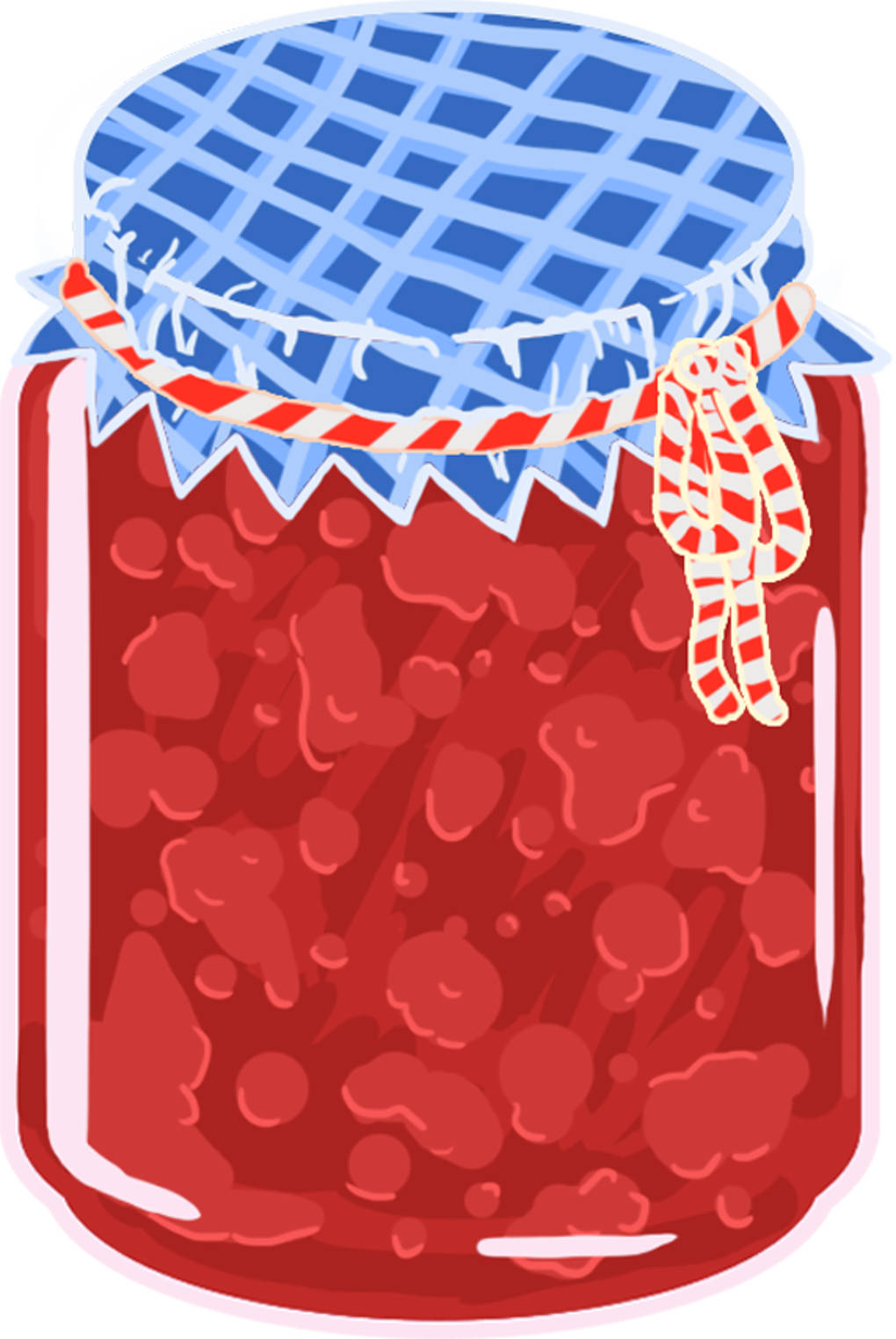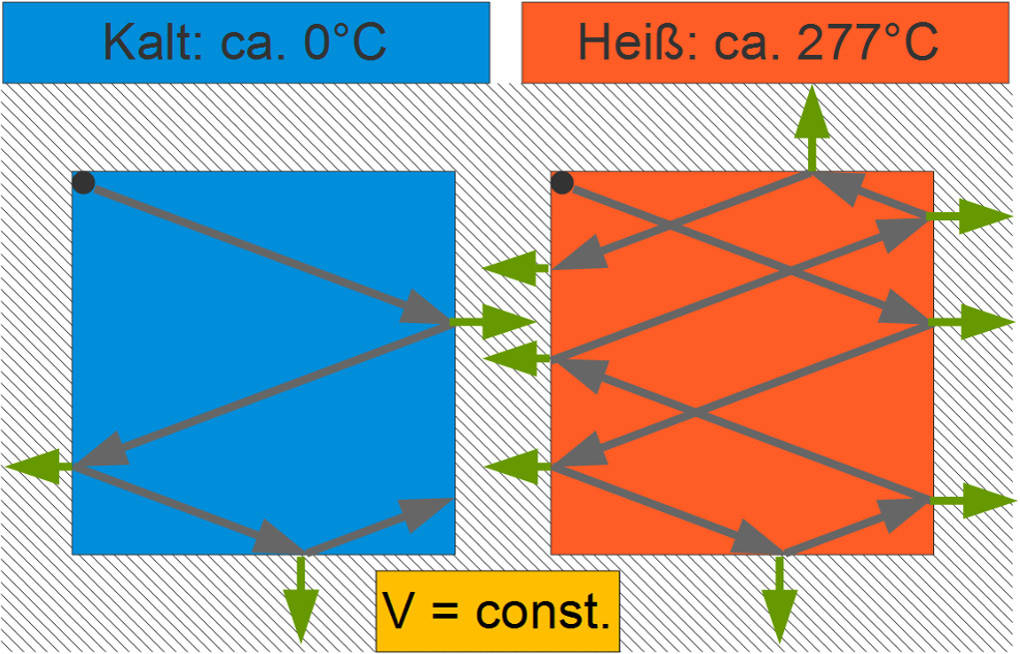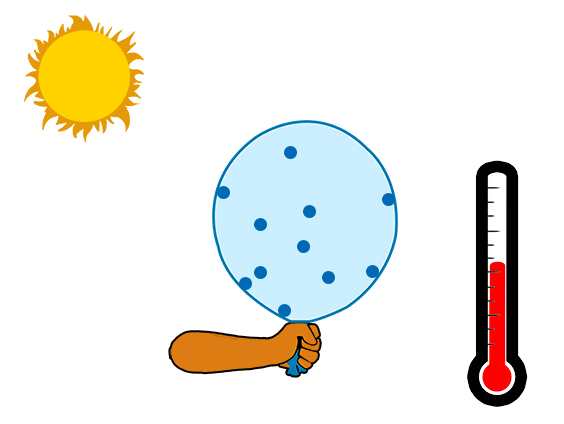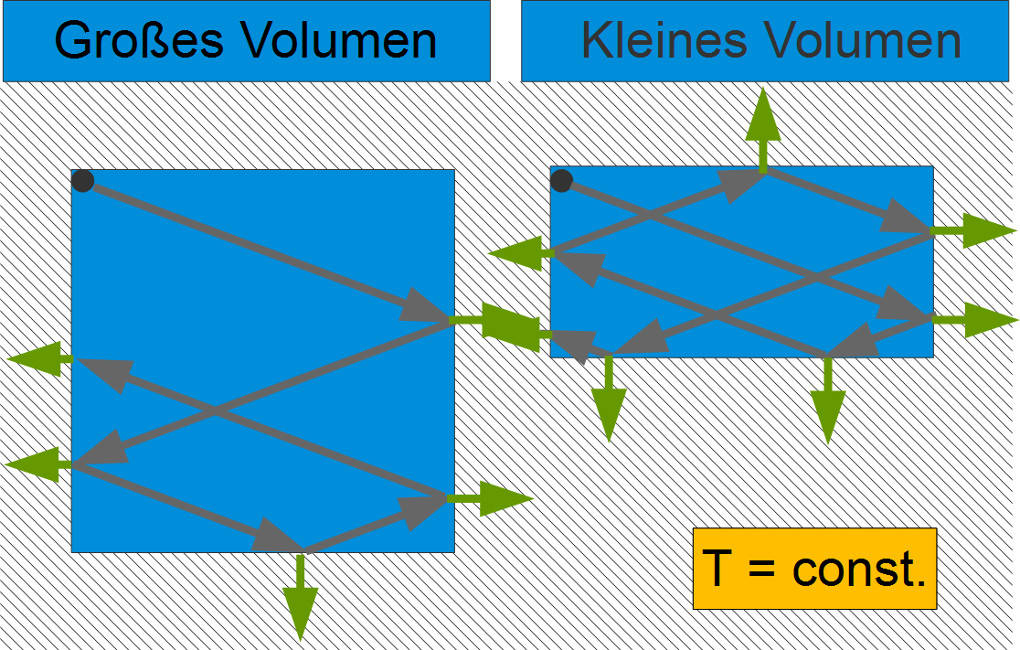# What are real and ideal gases

## The ideal gas and its laws

### What is an ideal gas? - Definition and overview

In nature, all substances are found in the three different Physical statesfirmly, liquid and gaseous in front. Gaseous substances are called gases. Examples of this are water vapor and air, which in turn is a gas mixture of nitrogen, oxygen, carbon dioxide and various noble gases such as helium.

All gases are actually real gases with a multitude of properties that make the formulas for an exact physical description complicated. As a rule, however, you can use the model of the ideal gas using the following simplifications:

• An ideal gas does not consist of atoms or molecules but of point particles without spatial expansion.

• In an ideal gas there are no inelastic collisions or other interactions between the particles. There are only elastic collisions between two particles or with the wall.

• The ideal gas does not change its amount of substance \$ n \$ i.e. the number of its gas particles \$ N \$. One speaks of one closed system.

There is no such thing as an ideal gas. However, if you dilute a real gas far enough, you can do it quite well with the simple one ideal gas law describe. This gives a connection between the State variablesprint, temperature and volume at. The temperature of a gas corresponds to im Particle model of matter the Kinetic energy of the gas particles. The hotter, the faster the particles move and the more force can be transferred to the walls of a vessel. You already have the relationship between the exerted force \$ F \$ and the area \$ A \$ as print got to know.

\$ p = \ frac {F} {A} \$

### The ideal gas law

An ideal gas is given by the formula

\$ \ frac {p \ cdot V} {T} = \ text {const.} \$

described. The product of pressure \$ p \$ and volume \$ V \$ divided by the temperature \$ T \$ is therefore a constant (const.). By holding on to one of the three state variables, you can find relationships between the other two variables and can thus use the ideal gas law derive further laws.

### Amontons' law

Now be \$ V \$ a constant volume. In reality, for example, it could be a Pressure bottle (Deodorant, hairspray etc.) or a Jar be. These vessels are firm and stable, their volume cannot be easily changed. In the formula of the ideal gas law, you can now do that Ignore volume \$ V \$, because it immutable is, and it remains:

\$ \ frac {p} {T} = \ text {const.} \$

left. So the quotient of pressure and temperature is constant. This relationship is known as that Law of Amontons.

The temperature drops from \$ T_0 \$ to \$ T_1 \$, the print from \$ p_0 \$ to \$ p_1 \$ sink and vice versa. So you get one linear relationship \$ p \ propto T \$ and the equation:

\$ \ frac {p_0} {p_1} = \ frac {T_0} {T_1} \$.

### Amontons' law in practice

If you put one Hairspray can in the sunso warmed up it and its content. Since that Constant volume remains, elevated also the print. In the worst case, the can can even explode as a result. Therefore, you should definitely keep them away from sources of heat. The opposite case occurs, for example, with Preserving jam a. The finished jam is poured very hot into a mason jar and sealed. Subsequently cools the contents of the glass from and the Pressure drops; it forms vacuum in the glass and it is difficult to open again. Because of this and because of the sugar in the jam, the jars have a long shelf life.### Representation in the particle model

In the particle model, you can faster Particles at the same time more power exert on the vessel wall than slower particles, as statistically they collide with it more often and also transfer more energy force with each impact.

Since the volume and thus the Area unchanged remains, follows from the increase in force bigger pressure.### Gay-Lussac's law

Now imagine a system with constant pressure before, for example one inflated balloon. Regardless of its size, there is always one Pressure equilibrium between the ambient pressure trying to compress the balloon and the pressure inside trying to inflate the balloon. The pressure in the balloon is always the same as the ambient pressure and therefore constant. In the ideal gas law you can now analogously to the above Neglect constant pressure \$ p \$ and you get:

\$ \ frac {V} {T} = \ text {const.} \$

So the quotient of volume and temperature is also constant and known as that Gay-Lussac's law.

### Gay-Lussac's law in practice

The temperature rises in a balloon from \$ T_0 \$ to \$ T_1 \$, for example if you put it in the Sun lays it must be Volume larger become. The balloon inflates from \$ V_0 \$ to \$ V_1 \$. The corresponding equation is:

\$ \ frac {T_0} {T_1} = \ frac {V_0} {V_1} \$.

### Representation in the particle model

In the particle model, you can fast particles more force exercise on the balloon wall. But at the same time the balloon and with it the Area growsremains the Constant pressure.### Boyle-Mariotte's Law

If you have the temperature \$ T \$ of a system constant hold, you can temperature in the formula of the ideal gas to neglect and the context remains

\$ p \ cdot V = \ text {const.} \$,

that as that Boyle-Mariotte's Law is known. The product of the pressure and the volume is constant. This means that the pressure increases from \$ p_0 \$ to \$ p_1 \$ if you reduce the volume from \$ V_0 \$ to \$ V_1 \$ and vice versa. You get one inversely proportional relationship \$ V \ propto \ frac1 {p} \$ and the equation:

\$ \ frac {p_0} {p_1} = \ frac {V_1} {V_0} \$.

### Boyle-Mariotte's Law in Practice

One with Sealed syringe filled with water is a good example. Are you trying to push the plunger in, so that volume to reduceso elevated the print. If the syringe were open, the water would now squirt out. That doing the Constant temperature remains is guaranteed if you press as slowly as possible. This is also known as adiabatic volume change.

### Representation in the particle model

The remains in the particle model Kinetic energy of the particles during the entire process equalsince we have the Temperature unchanged to let. Decreased one doing that volume, it follows from this that the particles collide with each other and the wall much more frequently, i.e. more power acts on the wall. Simultaneously decreased the surface and consequently increases the print.